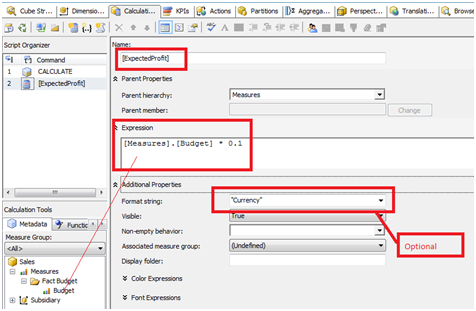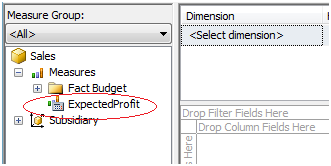## Thursday, January 24, 2013

### How to design calculated members in SSAS

1.     Background
The purpose of this document is to describe how to create Calculated Members in the cube and how to utilize it. Calculated member is very useful when we need to implement complex formulas/calculations in cube to achieve business requirements.

2.     What is Calculated Members and how to create it in Cube?
Calculated members are members of a dimension or a measure group that is defined based on a combination of cube data, arithmetic operators, numbers, and functions.

For example, you can create a calculated member that calculates the sum of two physical measures in the cube. Calculated member definitions are stored in cubes, but their values are calculated at query time.

Steps to create calculated member in the Cube:
• Open the Analysis Services solution for your cube
• Go to the Calculation tab of the solution then New Calculated Member
• It will open the window for creating new calculated member:

• Give the name of the new calculated member ‘Expected Profit’ and drag the measures from Calculation Tools and create a formula as per the business requirement.• Click on save button to Save the Calculated member.
• Process the cube and go to the ‘Browse’ tab of the cube. Here you can find the newly created calculated member ‘Expected profit’.• Check the data for the Calculated member:
• So we are able to see the data for the newly created calculated member.
• Custom calculation requirements can be achieved by using calculated member only.
• We can create complex expressions by combining data with arithmetic operators, numbers, and a variety of functions.
• Calculated members can be highlighted with colored fore/Background.